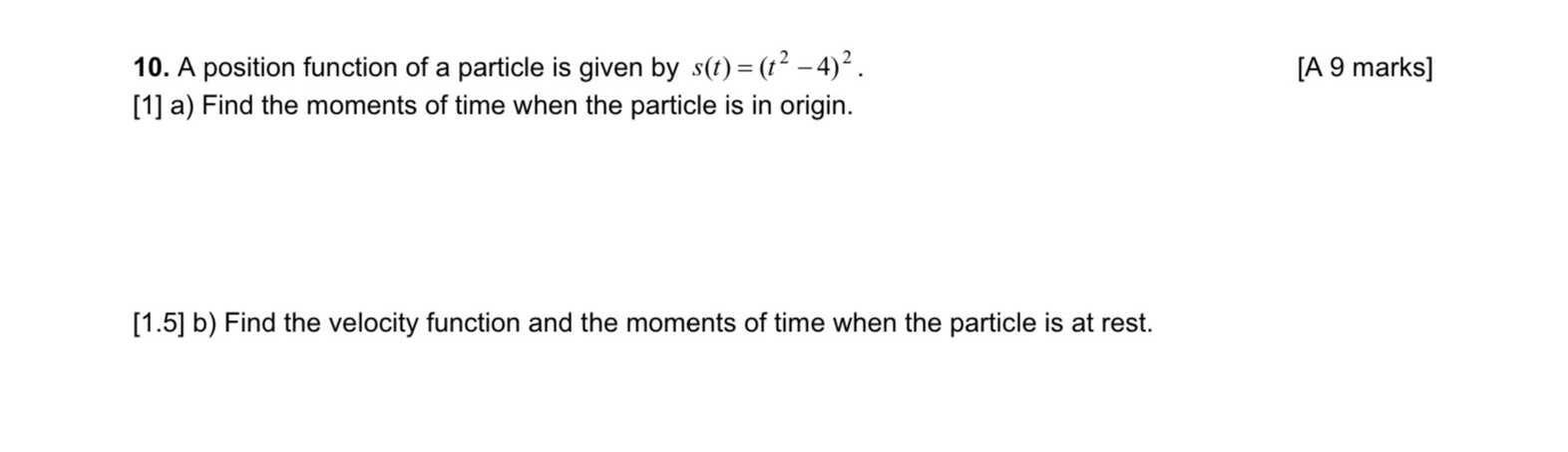### ¿Todavía tienes preguntas de matemáticas?

Pregunte a nuestros tutores expertos
Algebra
Pregunta10. A position function of a particle is given by $$s ( t ) = ( t ^ { 2 } - 4 ) ^ { 2 }$$ .

a) Find the moments of time when the particle is in origin.

b) Find the velocity function and the moments of time when the particle is at rest.

a) t = 2 sec , b) v(t) = $$4t(t^2- 4)$$